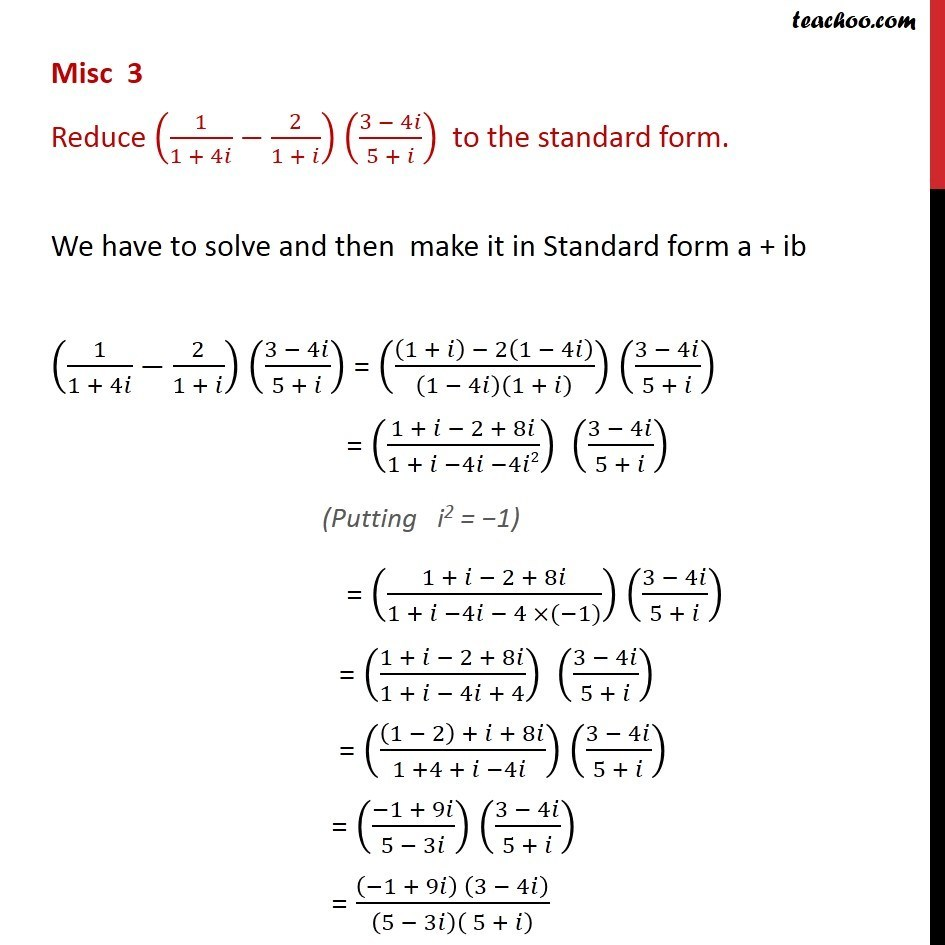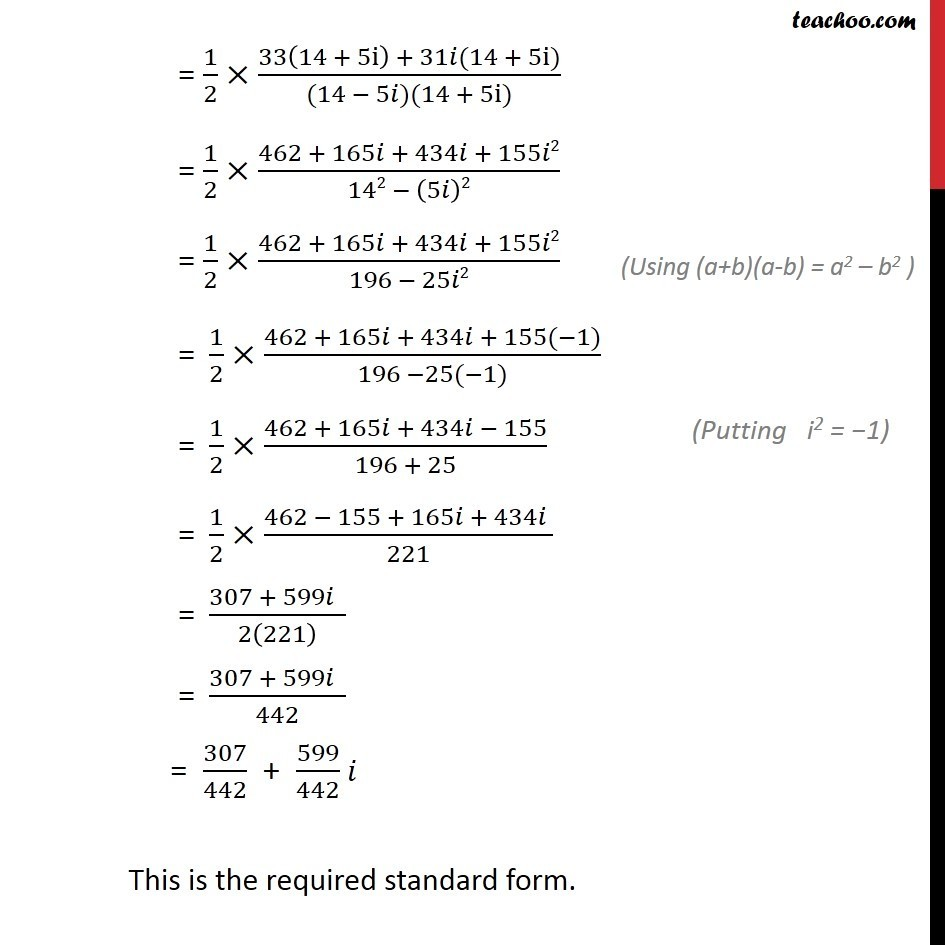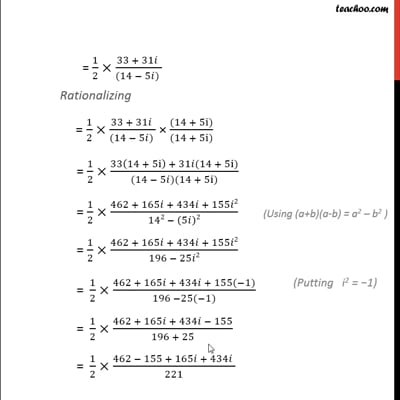Miscellaneous

Chapter 5 Class 11 Complex Numbers
Serial order wiseThis video is only available for Teachoo black users

Solve all your doubts with Teachoo Black (new monthly pack available now!)

### Transcript

Misc 3 Reduce (1/(1 + 4 ) 2/(1 + )) ((3 4 )/(5 + )) to the standard form. We have to solve and then make it in Standard form a + ib (1/(1 + 4 ) 2/(1 + )) ((3 4 )/(5 + )) = (((1 + ) 2(1 4 ))/(1 4 )(1 + ) ) ((3 4 )/(5 + )) = ((1 + 2 + 8 )/(1 + 4 4 2)) ((3 4 )/(5 + )) = ((1 + 2 + 8 )/(1 + 4 4 ( 1))) ((3 4 )/(5 + )) = ((1 + 2 + 8 )/(1 + 4 + 4)) ((3 4 )/(5 + )) = (((1 2) + + 8 )/(1 +4 + 4 )) ((3 4 )/(5 + )) = (( 1 + 9 )/(5 3 )) ((3 4 )/(5 + )) = (( 1 + 9 ) (3 4 ))/(5 3 )( 5 + ) = ( 1(3 4 ) + 9 (3 4 ))/(5 ( 5 + ) 3 ( 5 + ) ) = ( 3 + 4 +27 36 2)/(25 + 5 15 3 2) = ( 3 + 4 +27 36 1)/(25 + 5 15 3 1) = ( 3 + 4 +27 + 36)/(25 + 5 15 + 3) = ( 3 + 36+ 4 +27 )/(25 + 3 + 5 15 ) = (33 +31 )/(28 10 ) = (33 +31 )/(2(14 5 ) ) = 1/2 (33 +31 )/((14 5 ) ) Rationalizing = 1/2 (33 + 31 )/((14 5 ) ) ((14 + 5i))/((14 + 5i)) = 1/2 (33(14 + 5i) + 31 (14 + 5i))/((14 5 )(14 + 5i)) = 1/2 (462 + 165 + 434 + 155 2)/(142 (5 )2) = 1/2 (462 + 165 + 434 + 155 2)/(196 25 2) " = " 1/2 (462 + 165 + 434 + 155( 1))/(196 25( 1)) " = " 1/2 (462 + 165 + 434 155)/(196 + 25) " = " 1/2 (462 155 + 165 + 434 )/221 " = " (307 + 599 )/2(221) " = " (307 + 599 )/442 = 307/442 + 599/442 This is the required standard form.